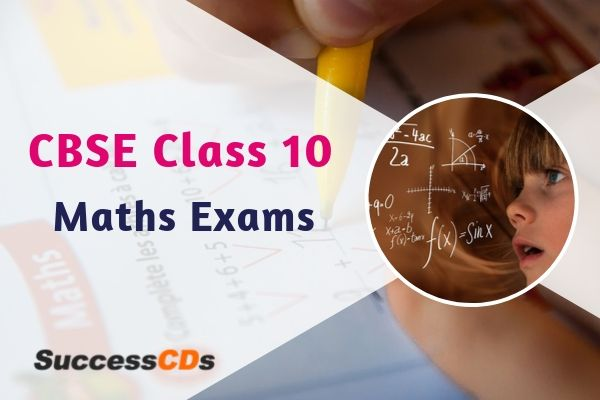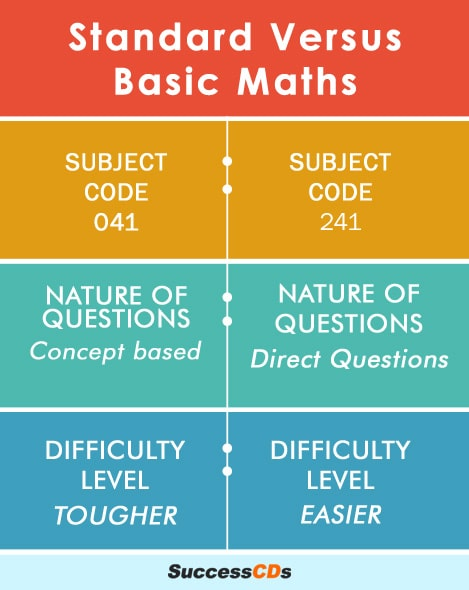Home >> CBSE >>

# CBSE Class 10 Maths Exams - Know the difference between the two PapersBy Gahna Arora

CBSE Class 10 Maths Exams - The Central Board of Secondary Education has come up with two exams for mathematicsin the year 2020 CBSE Board exams- Standard exam and the Basic exam. The basic exam is for those students who see mathematics as a subject that can be a hurdle in achieving their target score.

The CBSE has released Sample QP and MS for the Class 10 and Class 12 Board Exams 2020. Students can check out the Sample Question Papers and Marking Scheme for CBSE Board Exams for class X and XII, here

### Comparison between Mathematics Standard paper and Mathematics Basic paper

Let us see the points of difference between the Mathematics Standard exam and the Mathematics Basic exam

 Basis Standard Exam Basic Exam Subject codes 041 (same as the previous single Mathematics exam conducted by CBSE) 241 Nature of Questions Concept based More direct questions Difficulty level Tougher (similar to the previous single exam that was conducted) Easier Future prospects For those who want to pursue Mathematics in higher education For those who do not want to pursue Mathematics after Class 10

### Change in Syllabus of Class 10 Mathematics paper

The CBSE has not changed the syllabus of the class 10 Mathematics paper for any student regardless of whether they are appearing for Mathematics Standard exam (041) or the Mathematics Basic exam (241). All students will have to study the below mentioned 8 units that are a part of Mathematics Course Structure for Class 10. The only difference that will arise is in the difficulty level of the questions. Standard exam will consist of more concept based questions that are going to be higher in difficulty level as compared to questions in the Basic exam that are going to be direct questions.

Please see the latest syllabus for class 10 English Board exams here.
Please see the latest syllabus for class 10 Hindi Board exams here.

### Mathematics Course Structure for Class 10

The course structure of the Mathematics paper for class 10 along with the allotment of marks is as follows-

 S. No. Units Marks Number System 06 Algebra 20 Coordinate Geometry 06 Geometry 15 Trigonometry 12 6. Mensuration 10 7. Statistics and Probability 11 Total 80### Typology of Questions/ Difference in Question Paper Design

The weightage of marks for different types of questions will also differ in the Mathematics Standard exam and Mathematics Basic exam. It will be as follows-

 Type of Questions Description Weightage (marks) Standard Exam Basic Exam Remembering Basic Concepts 20 32 Understanding Interpretations revolving around the main idea 23 28 Applying Finding solutions to new situations 19 12 Analysing, Evaluating and creating Identifying, making judgements, giving opinions and providing alternative courses of action (possible solutions) 18 8 Total 80 80

However, if a student appears for the Basic exam, clears it but later on, changes his / her mind to pursue Mathematics further, then they have a way out. He / she can appear for the Mathematics Standard Compartment Exam that will be conducted by the CBSE in July 2020. Once the student clears the said exam, he can opt for Mathematics in higher classes as well.

Check out these useful tips to score 95% marks in English paper here.
Check out these useful tips to score 95% marks in Hindi paper here.
Check out these useful tips to score 95% marks in Sanskrit paper here.
Check out these useful tips to score 95% marks in Science paper here.
Check out these useful tips to score 95% marks in Social science paper here.

### Guidelines to help you choose the right type of exam for yourself

Our team of expert teachers have compiled these guidelines which will help students identify which exam should they opt for out of the two given options - Mathematics Standard exam or Mathematics Basic exam. These are as follows-

1. Do you want to pursue Mathematics in higher standards (11th onwards) ?

One cannot pursue Mathematics for higher education if one appears for the Basic Mathematics exam. If you want to pursue it for higher standards, you must opt for the

Mathematics Standard exam.

1. Are you not sure about your decision to not study Mathematics in class 11?

If you choose to appear for the Mathematics Basic exam (with a plan not to opt for Mathematics in class 11), but somehow, change your mind later. So, now you want to study Mathematics in classes 11 and 12, then you can appear for the Mathematics Standard Compartment Exam that will be conducted by the CBSE in July 2020.

1. Feel like mathematics isn’t for you? Does it make you reach your wit’s end every time you see a new problem?

If Mathematics is not your strongest forte and you are worried it might mess up with your overall score, it would be wise for you to appear for the Basic exam as it will ask more direct questions. Although the syllabus is the same, the difficulty level of both the examinations is varied, the Basic exam being on an easier level.

Hope that helps you make a wise decision about the suitable exam for you.

### Basic VS. Standard - Comparison between the questions.

As per the Sample Question Papers provided by the CBSE, check out this comparison between the type of questions that will be asked in the two papers from a given topic-

 Mathematics Basic exam Mathematics Standard exam 4 marks question Question: A metallic sphere of radius 4.2 cm is melted and recast into the shape of a cylinder of radius 6 cm. Find the height of the cylinder Solution: 4/3𝛱r3= 𝛱r2h 4/3(4.2)3= (6)2h =>h = 274/4100 Therefore, h = 2.744 cm Question: Water is flowing at the rate of 15km/hour through a pipe of diameter 14cm into a cuboidal pond which is 50m long and 44m wide. In what time will the level of water in the pond rise by 21cm? Solution: Quantity of water flowing through the pipe in 1 hour = 𝛱 7/100*7/100*15000m3 Required time =(50 *44 *21/100) /(𝛱*7/100*7/100*1500) = 2 hours 2 marks question Question: Two dice are thrown simultaneously at random. Find the probability of getting a sum of eight. Solution:  Of all those outcomes, the ones for which a + b = 8 are: 2+6, 3+5, 4+4, 5+3, 6+2 or 5 outcomes. P = 5/36 Question: Jayanti throws a pair of dice and records the product of the numbers appearing on the dice. Pihu throws 1 dice and records the squares the number that appears on it. Who has the better chance of getting the number 36? Justify? Solution: For Jayanti, Favourable outcome is (6,6) i.e, 1 Probability(getting the number 36) = 1/36 For Pihu, Favourable outcome is 6 i.e, 1 Probability(getting the number 36) = 1/6 ∴ Pihu has the better chance

From the above mentioned examples, it is clear that the Basic exam is a newly introduced concept which is relatively easier than the Standard exam which follows the traditional pattern of QP. The questions are direct and thus, can be solved with ease. The basic exam has been introduced for those students who do not wish to continue with Mathematics subject in higher classes. The aim of introducing the easier version of the Mathematics paper in class 10 board exams is that those students who fear Mathematics are not deterred and can perform well in the Board exams.